Fourier series representation

• I
Summary:
Trying to understand the requirements for a function to be represented by a Fourier series
Hi,

A function which could be represented using Fourier series should be periodic and bounded. I'd say that the function should also integrate to zero over its period ignoring the DC component.

For many functions area from -π to 0 cancels out the area from 0 to π. For example, Fourier series representation #1 below approximates such a function.

For some functions area from -π to -π/2 cancels out area from -π/2 to 0, and then area from 0 to π/2 gets cancelled by the area from π/2 to π. For example, Fourier series representation #2 below approximates such a function.

I'm not sure if the function needs to integrate to zero following these two patterns, or it should just amount to zero without actually following any pattern of area cancellation. Could you please let me know if I have it right?

Could you represent a function something like this using Fourier series? I'm just trying to get general concept about Fourier series right. Thank you for your help.

Fourier series representations #1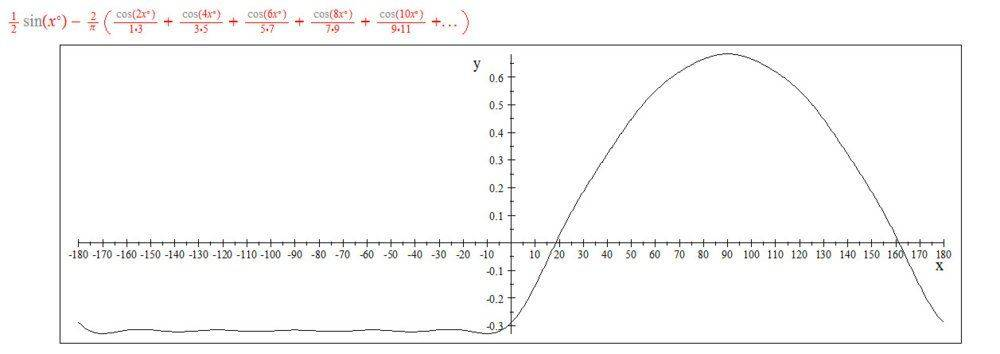Fourier series representations #2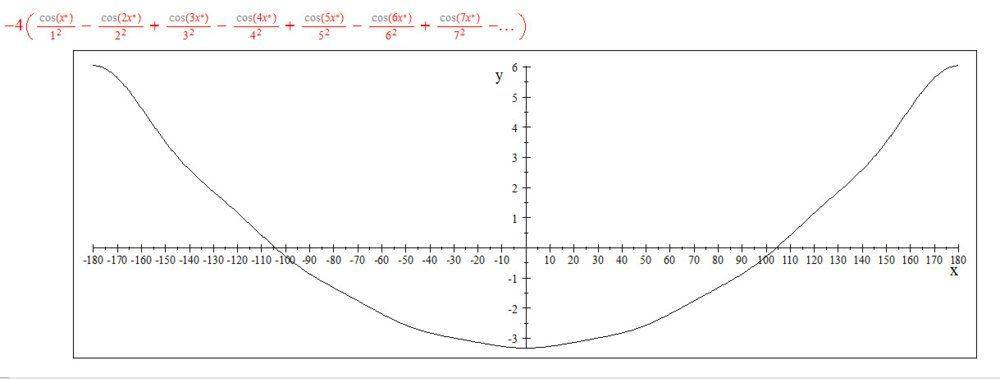Attachments

BvU
Homework Helper
I'd say that the function should also integrate to zero over its period ignoring the DC component.
That's a way of saying twice the same -- the DC component IS (proportional to) the integral over the period. And a Fourier series starts with the coefficient for ##\cos(0)## -- a constant.

 and, to answer your question: yes, your slightly pathological function can also be represented by a Fourier seeries

•PainterGuy
Thank you!

But doesn't there exist a periodic function(s) which doesn't integrate to zero over its period? Thanks a lot for your help.

BvU
Homework Helper
You can add a constant to any periodic function and it remains periodic. And the only term that changes in the Fourier series is the ##a_0## term.
Your question is very strange for me.

•PainterGuy
Thank you!

Yes, you are right it was a silly one! :)

RPinPA
Homework Helper
The original function doesn't need to be periodic. The more general application is that you want to represent the function on an interval [a, b], and you don't care about outside the interval.

The range of functions which can be approximated by a Fourier series on an interval is pretty broad. You can for instance have a jump discontinuity as in a step function. The series doesn't converge to f(x) at the point of discontinuity (for instance if you have a jump from 0 to 1 at x0, the series may converge to 1/2).

I don't recall the precise conditions for pointwise convergence of a Fourier series, but they're mentioned in this thread:
https://math.stackexchange.com/ques...nction-can-be-represented-as-a-fourier-series "The deeper fact is Carleson's theorem, which was one of the most difficult achievements in 20th century analysis, and tells us about the precise conditions for pointwise (actually, "pointwise almost everywhere") convergence of Fourier series"

•PainterGuy and DrClaude
Hi,

I had few related questions to this discussion so I thought it'd better to ask those here.

Question 1: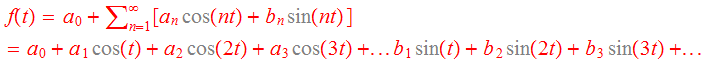A sinusoid, e.g. cosine, is mostly given as
cos(θ)=cos(ωt)
where ω=2πf

In Fourier series a sinusoid is mostly written as:
cos(nt)
If n=1 then,
cos(1⋅t)=cos(ω⋅t)
⇒1⋅t=ω⋅t
⇒ω=1
⇒2πf=1
⇒f=(1/(2π))= 0.15915

It would mean that for Fourier series of any function the starting frequency would be 0.15915 Hz but why isn't the starting frequency "1 Hz"?

Question 2:
I prefer trigonometric form of Fourier transform over the exponential form because it's easier to think of it as an extension of trigonometric form Fourier series. Given below are two excerpts about trigonometric form of Fourier transform.

Excerpt #1: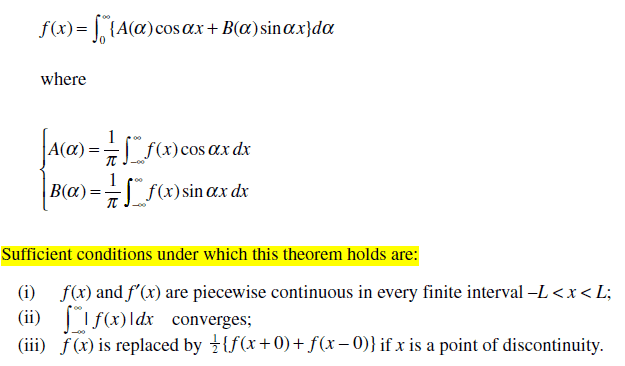Excerpt #2: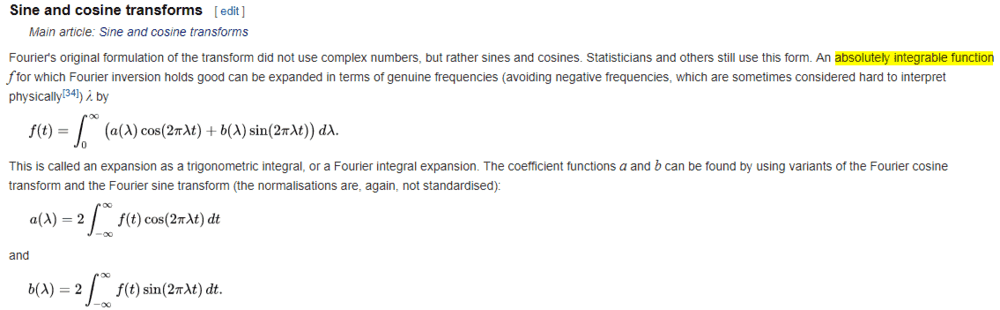Source: https://en.wikipedia.org/wiki/Fourier_transform#Sine_and_cosine_transforms

The given below Fourier transform for a unit pulse is found using exponential form of Fourier transform. Is it possible to find it using trigonometric form of Fourier transform? I'm sorry I didn't do it but it looks like it's not possible. Although considering the sufficient conditions given in excerpt #1 above, the f(t) is a piecewise continuous function.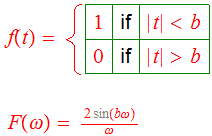Thank you for your help and time.

RPinPA
Homework Helper
Question 1: That's not true. The series you give will reproduce a function which has a period of ##2\pi## seconds or which is defined on an interval of width ##2\pi## seconds, such as ##[0,2\pi]## or ##[-\pi,\pi]##

In general a function with period ##T## or defined on an interval of width ##T## will be represented by a series with fundamental frequency ##f = 1/T##, i.e. sums of ##\cos(2\pi n t/T)## and ##\sin(2\pi nt/T)##.

It would mean that for Fourier series of any function the starting frequency would be 0.15915 Hz but why isn't the starting frequency "1 Hz"?

It would mean that the Fourier series of a function with period ##2 \pi## seconds was composed of sinusoids which are periodic over ##2\pi## seconds. Not 1 Hz because 1 Hz does not repeat itself in ##2 \pi## seconds.

In Question 2 you're looking at the continuous Fourier Transform, which is generalized from the series version. The series version as I said is appropriate for periodic functions or functions which are defined only over a finite interval. The continuous transform is defined for a different class of functions which are given in your excerpt.

They're not quite the same though obviously there's a connection.

Anyway in answer to your question, your ##f(t)## is an even function. That causes the integral for ##b(\lambda)## to be 0 for all ##\lambda##. The integral for ##a(\lambda)## just becomes an integral of ##\cos(2\pi\lambda t)## from ##-b## to ##+b## which gives the expression you're looking for.

•PainterGuy
RPinPA
Homework Helper
Here are the integrals.
$$\int_{-\infty}^\infty f(t) \cos(2\pi \lambda t) dt = \int_{-b}^b \cos(2\pi \lambda t) dt \\ = \frac {1}{2\pi\lambda} \left [\sin(2\pi\lambda b) - \sin(-2\pi\lambda b) \right ] \\ = \frac {1}{2\pi\lambda} \left [\sin(2\pi\lambda b) + \sin(2\pi\lambda b) \right ] \\ = \frac {2 \sin(2 \pi \lambda b)} {2 \pi \lambda} \\ = \frac {2 \sin(\omega b)} {\omega} \text{ where } \omega = 2\pi\lambda$$

$$\int_{-\infty}^\infty f(t) \sin(2\pi \lambda t) dt = \int_{-b}^b \sin(2\pi \lambda t) dt \\ = \frac {1}{2\pi\lambda} \left [-\cos(2\pi\lambda b) + \cos(-2\pi\lambda b) \right ] \\ = \frac {1}{2\pi\lambda} \left [-\cos(2\pi\lambda b) + \cos(2\pi\lambda b) \right ] = 0$$

Last edited:
•PainterGuy
This is a far more difficult question to answer completely than it may appear. It can be answered incompletely, including the following.
First suppose ##\int_0^{2\pi} |f(x)|^2\,dx < \infty.## (The absolute value sign is needed since ##f(x)## need not be a real number; it is a complex number, so its square need not be positive or even real.) That is enough to guarantee it has a Fourier series in which the sum of the squares of the absolute values of the coefficients is the same as the foregoing integral. But does the series converge to ##f(x)##? In one sense it does: $$\lim_{n\to\infty} \left| f(x) - \sum_{k=-n}^n c_k e^{ikx}\right|^2 = 0.$$ But that falls short of saying that for every number ##x## you have $$\lim_{n\to\infty} \sum_{k=-n}^n c_k e^{ikx} = f(x) \qquad \text{(?)}$$ It was not until the 1960s that it was shown that for almost every value of ##x## that is true, and "almost every" means the measure of the set of exceptions is zero. That means that no matter how tiny you make a positive number ##\varepsilon,## the set of exceptions fits within a union of open intervals the sum of whose lengths is no more than ##\varepsilon.##

•PainterGuy
Question 1: That's not true. The series you give will reproduce a function which has a period of ##2\pi## seconds or which is defined on an interval of width ##2\pi## seconds, such as ##[0,2\pi]## or ##[-\pi,\pi]##

In general a function with period ##T## or defined on an interval of width ##T## will be represented by a series with fundamental frequency ##f = 1/T##, i.e. sums of ##\cos(2\pi n t/T)## and ##\sin(2\pi nt/T)##.

I believe that I understand it now. Actually "t" or "x" along the x-axis doesn't just represent time. Any periodic phenomenon could be stated in terms of degrees where 360° stands for one complete cycle.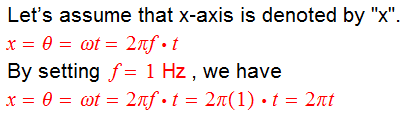So, "x" is implicitly given in terms of "2πt".
When t=0 seconds: x=0°.
When t=0.5 seconds: x=180°.
When t=1 seconds: x=360°.
When t=2 seconds: x=720°.

I'm sorry if I'm still having it wrong.

Note to self: If you are measuring two periodic phenomena along the same axis, a slower phenomenon would take 360° to complete one period and the other faster phenomenon having double the frequency of slower phenomenon might apparently 'seem' to take just 180° but the faster phenomenon is also taking 360° to complete its period; the "180°" just mean that the second require comparatively just 180° of the slower phenomenon to complete its period.

It would mean that the Fourier series of a function with period ##2 \pi## seconds was composed of sinusoids which are periodic over ##2\pi## seconds.

I'm sorry to split hairs, but wouldn't only fundamental frequency be periodic over 2π seconds and the harmonics would be periodic over multiples of 2π seconds?

Here are the integrals.
$$\int_{-\infty}^\infty f(t) \cos(2\pi \lambda t) dt = \int_{-b}^b \cos(2\pi \lambda t) dt \\ = \frac {1}{2\pi\lambda} \left [\sin(2\pi\lambda b) - \sin(-2\pi\lambda b) \right ] \\ = \frac {1}{2\pi\lambda} \left [\sin(2\pi\lambda b) + \sin(2\pi\lambda b) \right ] \\ = \frac {2 \sin(2 \pi \lambda b)} {2 \pi \lambda} \\ = \frac {2 \sin(\omega b)} {\omega} \text{ where } \omega = 2\pi\lambda$$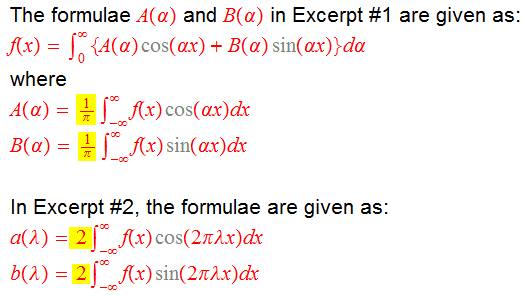In your calculation, you used formulae from Excerpt #2. You didn't use the factor "2" in front of integral.

I did the calculation using formulae from Excerpt #1 and didn't use the factor of (1/π). I reached the same solution as you and apparently this is the correct solution. Then, why would Excerpt #1 and #2 have those factors?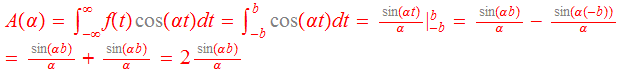The most used form of Fourier transform is exponential form given as: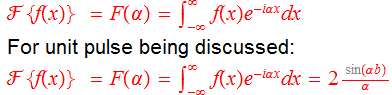By comparing trigonometric form and exponential form, we can see that A(α)=F(α).

Both A(α) and F(α) give us the magnitudes of involved frequencies and it implicitly means that if you add all the frequencies with given magnitudes, you would get f(x) back.

On the other hand, trigonometric form does convey the information by explicitly stating how to get f(x) back as shown below.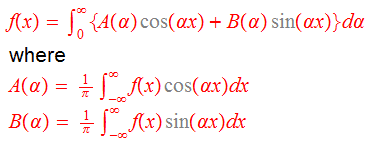I was trying to find that why the exponential form of Fourier transform is more popular compared to its trigonometric equivalent. I was able to find an answer, https://math.stackexchange.com/ques...er-series-versus-trigonometric-fourier-series, which gives the reasons in terms of exponential form of Fourier series and trigonometric form of Fourier series. It has more to do with cleanliness, compactness, and easy manipulation of exponential form compared to the trigonometric and it wouldn't be wrong to say that both are equally applicable mathematically.

Thanks a lot for your help and time!

wouldn't only fundamental frequency be periodic over 2π seconds and the harmonics would be periodic over multiples of ##2\pi## seconds?

No, that's backwards. The harmonics would be over submultiples, i.e. divisors, of ##2\pi##, i.e. ##2\pi/2,\, 2\pi/3,\, 2\pi/4,\, 2\pi/5,\,\ldots## They have higher frequencies, hence shorter periods. Thus all of them would have ##2\pi## as a period, but not necessarily as a shortest period.

•PainterGuy
Hi

Check the dirichlet conditions
Any function that satisfies these, has a fourier series rep.

Hi,

It was not until the 1960s that it was shown that for almost every value of xxx that is true, and "almost every" means the measure of the set of exceptions is zero. That means that no matter how tiny you make a positive number ε,ε,\varepsilon, the set of exceptions fits within a union of open intervals the sum of whose lengths is no more than ε.

Thank you. This was also mentioned in post #6; see the quote below.

"The deeper fact is Carleson's theorem, which was one of the most difficult achievements in 20th century analysis, and tells us about the precise conditions for pointwise (actually, "pointwise almost everywhere") convergence of Fourier series"

Wikipedia article: https://en.wikipedia.org/wiki/Carleson's_theorem

Hi

Check the dirichlet conditions
Any function that satisfies these, has a fourier series rep.

Thanks. I agree with you.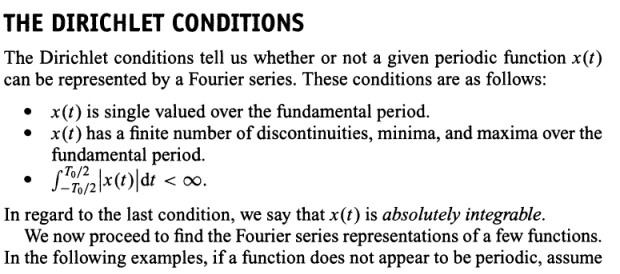Hi again,

I'm sorry that the questions below aren't that clear but I don't really know how to put them any other way.

Fourier transform (or series) could be represented in two forms, exponential form or trigonometric form, as is shown below.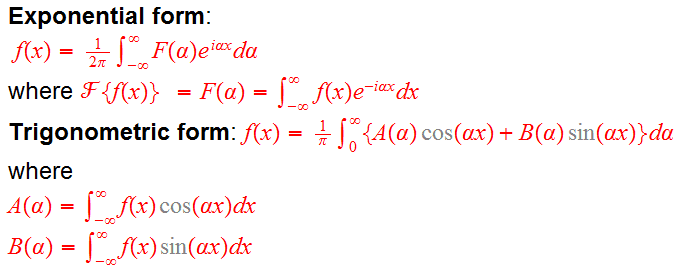Exponential form uses all the frequencies from -∞ to +∞; in other words it involves negative frequencies which many people, including me, find quite weird but it doesn't make sense to ask the question again that 'what are negative frequencies' when it has already been asked many, many times at many, many different places. On the other hand, trigonometric form of Fourier transform, which isn't used very often does not use negative frequencies.

Well, one question does come to mind that which of the two between negative and positive frequencies, are more real 'physically and practically'. I'm not even sure if it's a legit question. The answer could be that mathematically exponential form is superior because it's symmetric around the origin.

This is another related question. Suppose that the frequency spectrum of a modulating signal is found using the trigonometric form of Fourier transform, and, say, this spectrum extends from 0 Hz to 500 Hz. Now let's suppose that frequency of carrier wave is 2500 Hz. As there are no negative frequencies in the modulating signal therefore only upper side band (USB) should be generated and there should be no lower side band (LSB). But I have never seen any picture of a spectrum of modulated signal, such as AM, where LSB is missing; mostly modulated signal appears like this. Why isn't it possible to avoid LSB when using only positive frequencies, or is it just me?

Thank you for the help!

Attachments

RPinPA
Homework Helper
As there are no negative frequencies in the modulating signal therefore only upper side band (USB) should be generated

That's incorrect. Having only one frequency at baseband doesn't mean you'll have only one frequency after mixing up. Let's analyze a simple amplitude modulated carrier wave.

Let's say the carrier frequency is ##\Omega## (I'm going to use angular frequencies such as ##\Omega = 2\pi F## to avoid writing lots of ##2 \pi's##) so the carrier wave is ##\sin(\Omega t)##.

Now we modulate it at frequency ##\omega## so our signal is ##s(t) = \sin(\omega t) \sin(\Omega t)##

Let's derive a little trig identity that we'll need. Consider ##\cos(x + y) = \cos(x) \cos(y) - \sin(x) \sin(y)## and ##\cos(x - y) = \cos(x) \cos(y) + \sin(x) \sin(y)##. So ##\cos(x - y) - \cos(x + y) = 2\sin(x) \sin(y)##

Then ##s(t) = (1/2) \left [ \cos(\Omega - \omega)t - \cos(\Omega + \omega)t \right ]##

Mixing (multiplying by a sinusoid) produces both sum and difference frequencies, and removing one of those frequencies requires an extra filtering step.

•PainterGuy
Thank you for correcting me.

For some reason I was wrongly under the impression that lower side band is a result of using negative frequencies of the exponential form.

I understand that mathematically it's more practical to use the exponential form of Fourier transform. But at the same time one can do every calculation using the trigonometric form which only uses 'more sensible' positive frequencies and end up with the same result as with the exponential form. Now the question is why all this confusion about the 'physical significance' of negative frequencies is so important. A complex sinusoid, e^iωt or e^iθ, is mostly thought of as a counterclockwise rotating wheel. If that wheel can theoretically rotate one way then why not the other way in clockwise direction. In other words, it's like the flipping of a coin where the probability for both sides is equal, i.e. 1/2, and 1/2 + 1/2 =1. We know that the frequency spectrum found using exponential form is symmetrical around the y-axis, and the magnitudes of corresponding positive and negative frequencies are added to get full magnitude. This adding up of magnitudes is much like adding "1/2" probability of a coin to get "1". In short, negative frequencies are a mathematical construct or abstraction to provide symmetry.

I have also read about time going backwards in case of negative frequencies and going forward in case of positive frequencies. In the expression below, integral from -∞ to 0 involve negative frequencies because you need to sum up sinusoids made up of negative frequencies. Why don't we just say that "ω" is a kind of vector where +ω represents counterclockwise direction and -ω shows clockwise direction? I understand that strictly speaking calling "ω" a vector is a lame statement! (Edit: "ω" could be called a signed scalar just like +θ is considered being measured counterclockwise from the positive x-axis and -θ is the angle measured clockwise; θ=ωt so it could be said that -θ={-ω}t) But saying time going backwards is also a little bit of science fiction. Could you please let me know your opinion about this negative frequencies confusion, or do you think you could make it easier for me to understand? Thanks.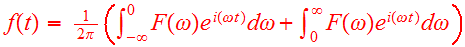Please have a look on the attachment, fourier_expo1.

I'm not sure how the author gets to step 16. I tried it but "-" sign stands in my way as shown below.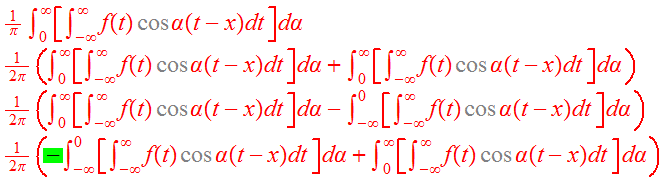Thank you for your help and time!

Attachments

Last edited:
RPinPA
Homework Helper
This adding up of magnitudes is much like adding "1/2" probability of a coin to get "1". In short, negative frequencies are a mathematical construct or abstraction to provide symmetry.

I can see why you prefer the trigonometric form and are a little distrustful of the complex form. These are representations of real signals. As such, they only have positive frequencies, and their value better turn out to be real.

The exponential form is much easier to deal with mathematically, but it can potentially lead to complex solutions. The reason there are negative frequencies, in my view, is simply because when we are constructing a real signal, every complex number must also be paired with its complex conjugate. Both the number and its conjugate must be present and added.

The negative frequencies add zero information when they arise from the transformation of a real signal. They don't have a physical meaning.

Another way I think of it is that there are two pieces of independent information needed to completely reconstruct a real signal. In the complex transform, they are contained in the real and imaginary parts of the transform (at positive frequencies). In the trigonometric version, they are the sine and cosine transforms.

Why don't we just say that "ω" is a kind of vector where +ω represents counterclockwise direction and -ω shows clockwise direction?

I'm sorry but I don't really follow what point you're making in this paragraph.

A real-valued signal consisting of a modulated carrier wave can be thought of as having an instantaneous magnitude and phase relative to the carrier, that is as ##A(t) \sin [\Omega t + \phi(t)]##. Again, two pieces of information needed to describe it. In actual receiver logic I've often seen that what is measured is something like a sine and cosine transform which are then treated as the real and imaginary parts of the corresponding complex number.

When you transform to baseband, subtracting off the carrier frequency, you have an actual complex-valued signal which doesn't have conjugate symmetry. The negative frequencies have real physical meaning. But nothing exotic: what they really mean is a signal whose instantaneous frequency is less than the carrier. When you go the other way to put a complex modulation on a transmitted carrier, you're using real-valued amplitudes and phases. There's nothing actually complex or at "negative frequency" here.

I guess what I'm saying about negative frequencies is don't worry about it. Either think of them as a mathematical artifact from taking a complex transform of a real-valued thing, or think of them as relative to the carrier.

•PainterGuy
RPinPA
Homework Helper
Please have a look on the attachment, fourier_expo1.

I'm not sure how the author gets to step 16. I tried it but "-" sign stands in my way as shown below.

View attachment 246883

Thank you for your help and time!

The author says the integrand is an even function of ##\alpha##, so ##\int_{-\infty}^0 d\alpha## should be the same as ##\int_{0}^{\infty} d\alpha##. You have a sign error in your third line.

Let's define ##g(\alpha) = \int_{-\infty}^{\infty} f(t) \cos \alpha(t - x) dt##. Then ##g(-\alpha) = g(\alpha)## because ##\cos## is even, i.e., ##\cos [-\alpha(t - x)] = \cos \alpha(t - x)##.

So ##\int_{0}^{\infty} g(\alpha) d\alpha## = ##\int_{0}^{\infty} g(-\alpha) d\alpha## = -##\int_{0}^{-\infty} g(\beta) d\beta## where ##\beta = -\alpha, d\beta = -d\alpha##

Thus ##\int_{0}^{\infty} g(\alpha) d\alpha## = ##\int_{-\infty}^0 g(\beta) d\beta##

Intuitively, if you have an even function, so the graph to the left of the y-axis is the mirror image of the graph to the right of the y-axis, then the area under the left half should be the same as the area under the right half.

•PainterGuy

The author says the integrand is an even function of αα\alpha, so ∫0−∞dα∫−∞0dα\int_{-\infty}^0 d\alpha should be the same as ∫∞0dα∫0∞dα\int_{0}^{\infty} d\alpha. You have a sign error in your third line.

I did read that statement about the integrand being an even function. In case of an even function, Fourier sine term is zero, and in case of odd function the cosine term is zero.

I understand what you said about integral of an even function in general but in this specific case I'm confused.

I believe the author is saying, like you, that the part in yellow results in an even function of α.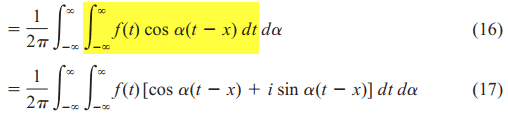Let's define g(α)=∫∞−∞f(t)cosα(t−x)dtg(α)=∫−∞∞f(t)cos⁡α(t−x)dtg(\alpha) = \int_{-\infty}^{\infty} f(t) \cos \alpha(t - x) dt. Then g(−α)=g(α)g(−α)=g(α)g(-\alpha) = g(\alpha) because coscos\cos is even, i.e., cos[−α(t−x)]=cosα(t−x)cos⁡[−α(t−x)]=cos⁡α(t−x)\cos [-\alpha(t - x)] = \cos \alpha(t - x).

I agree that cosα(t-x) is an even function but the expression also involves f(t) and I don't think we really know if it's even or odd or both. Also, g(α) is an integral expression where integration variable is time and not α. My confusion stems from this point.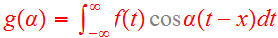The product of two even functions is an even function. The product of two odd functions is an even function. The product of an even function and an odd function is an odd function.

For example, in this thread, https://www.physicsforums.com/threads/ambiguous-results-for-two-fourier-transform-techniques.974660/ , Fourier transform of f(t)=a.e^(-bt).u(t) was found to be a/(b+jω) or a/(b+jα); u(t) is a step function. The plot shown below is for a=b=1.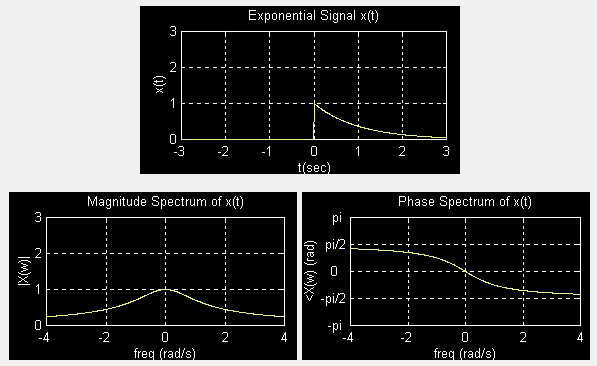Source: http://pages.jh.edu/~signals/spectra/spectra.html

The magnitude of 1/(1+jα) is an even function but the phase is an odd expression. This, 1/(1+jα), function is same as g(α) or equivalent to the expression in yellow highlight.

So is g(α) or g(ω) an even function in this case?

Where am I going wrong? Could you please guide me?

Attachments

Last edited:
RPinPA
Homework Helper
I agree that cosα(t-x) is an even function but the expression also involves f(t)

Which is independent of ##\alpha##, and therefore is unaffected when you change ##\alpha## to ##-\alpha##. When you make that change, the equation for ##g(\alpha)## is completely unchanged and therefore results in exactly the same function. The question of whether ##g(\alpha)## is even is in terms of that change, relative to an integral over ##\alpha##. The only question is what happens to it when you change ##\alpha## to ##-\alpha##.

Also, g(α) is an integral expression where integration variable is time and not α. My confusion stems from this point.

##t## is a dummy variable in ##g(\alpha)##. After you perform the integration, there is no ##t## there, which is why you can write ##g## as a function of ##\alpha## with no dependence on ##t##. There is no ##t##. You could call it ##x##. You could call it ##s##. You could call it anything you want, but whatever you call it, it no longer appears after you do the integration.

Last edited:
•PainterGuy
RPinPA
Homework Helper
Here is a simpler example of what's happening here.

Define ##g(\alpha) = \int_1^2 (\alpha t)^2 dt##. That may look like a function of ##t## to you, but it's not. ##t## is a dummy variable which does not exist outside the integral sign. We can explicitly calculate the value of ##g(\alpha)## by doing the integral.
##g(\alpha) = \alpha^2 \int_1^2 t^2 dt = \alpha^2 (2^2 - 1^3) = 3\alpha^2## and now you can see explicitly that (1) ##g## does not depend on ##t## and (2) ##g## is an even function of ##\alpha## and (3) it doesn't make sense to ask whether ##g## is an even function of ##t## or an odd function of ##t## because it is not a function of ##t## at all.

That's happening in your expression. The integral of ##g(\alpha)## when ##\alpha## goes from ##0## to ##\infty## is exactly the same as the integral of ##g(\alpha)## when ##\alpha## goes from ##-\infty## to ##0## because ##g(\alpha)## is a function of ##\alpha## which is unchanged when ##\alpha## is changed to ##-\alpha##. No matter what ##f(t)## is, ##g(\alpha)## does not contain a ##t##.

But even if it did contain other variables, the only thing that matters in the question of whether ##g## is even with respect to ##\alpha## is what happens when you change ##\alpha## to ##-\alpha##.

•PainterGuy
Thanks a lot for your help! I really appreciate it.

The following is a note to myself or for someone else like me who stumble upon this thread.

The author said, "We note that (16) follows from the fact that the integrand is an even function of a. In
(17) we have simply added zero to the integrand; ..., because the integrand is an odd function of a.
"

Given below is precise and straightforward answer.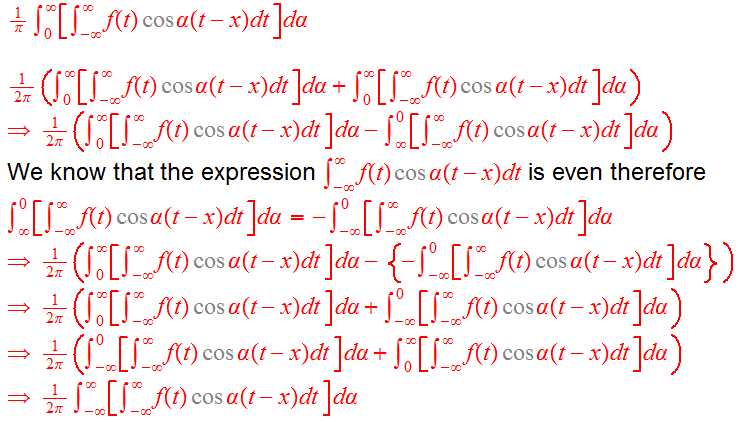We can probe it further to understand it better. Let's discuss a particular case, f(t), which resembles in the original expression being discussed. We are going to discuss Riemann sum too.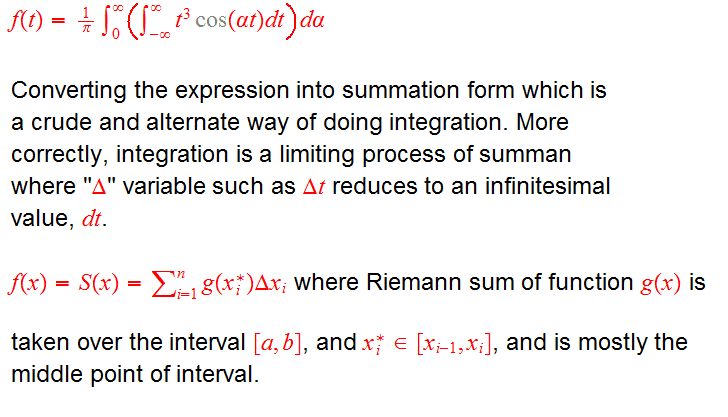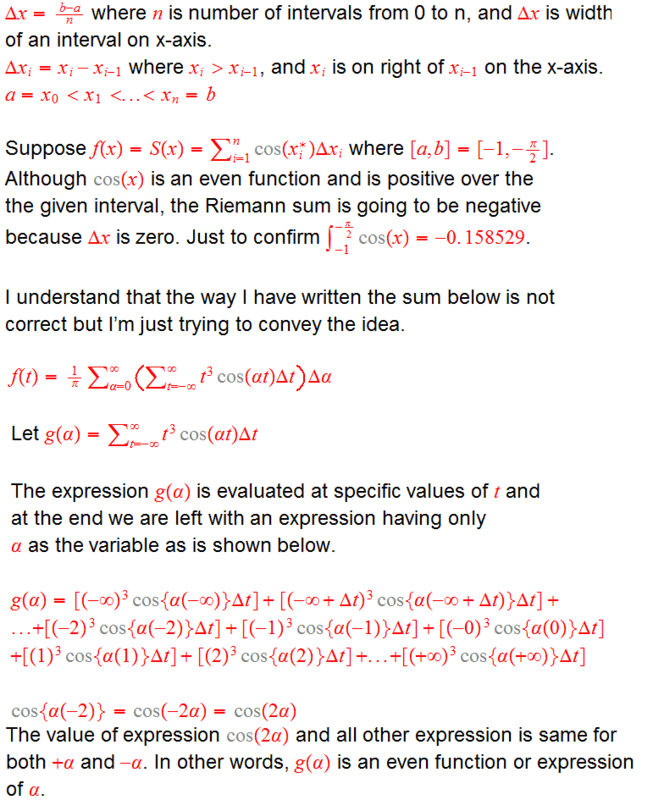Now let's evaluate the same expression analytically.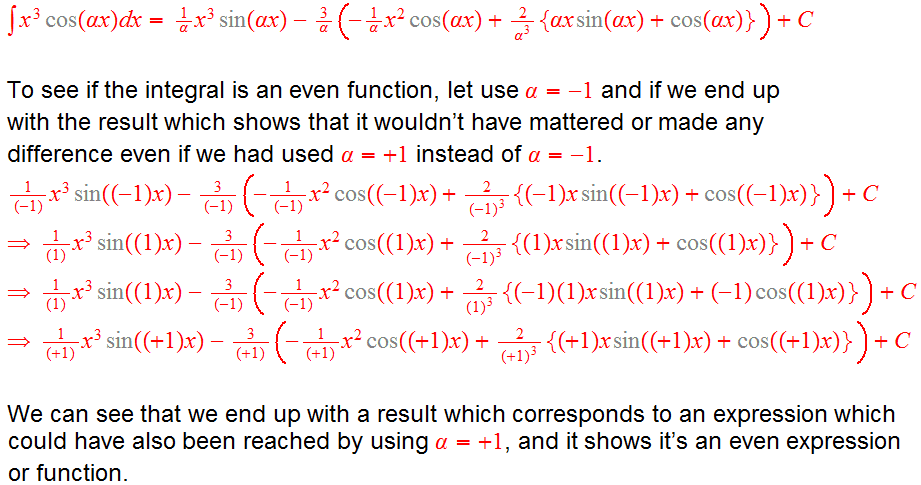Now let's focus on this part where the author said, "In (17) we have simply added zero to the integrand; ..., because the integrand is an odd function of a."

In the following calculation everything seems to cancel out but those differing "+" and "-" signs wouldn't let the expression cancel completely. Both signs should have been either "+" or "-". I wasn't able to track the error. The integral was evaluated using Symbolab.

I evaluated the same expression using TI-89 and the expression does cancel out to give "0" as said by the author. The TI-89 calculation is also shown.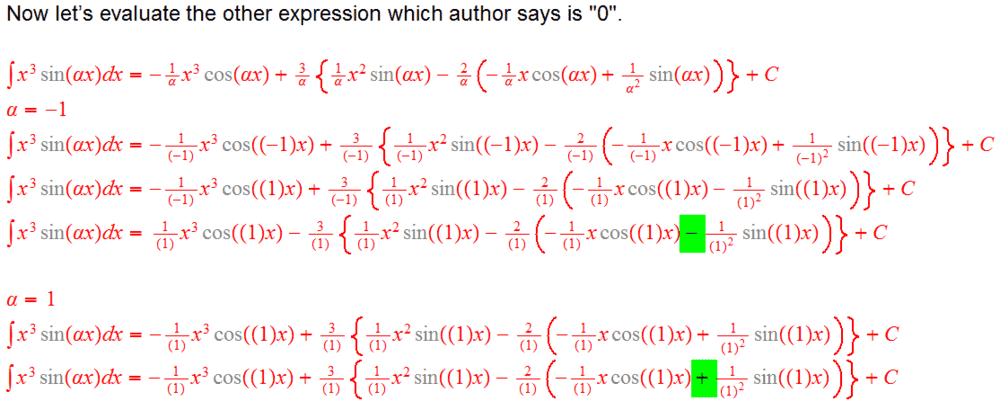This post continues into the next posting.

Last edited: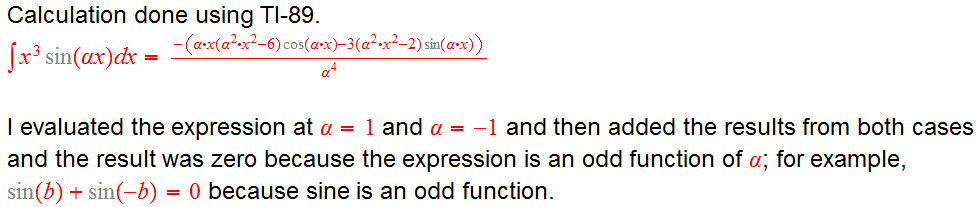Question:
By the way, let's say F(x)=∫f(x)dx. I understand that an integral is always evaluated using two limits like this this: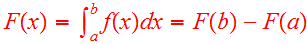Does it mean anything when an integral is evaluated over only a single limit, like this F(b)? I understand that it'd given us a numeric value but does this numeric value mean anything?

Thank you for your help and time!